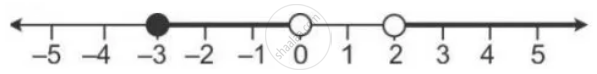Share

# Illustrate the Set {X: -3 ≤ X < 0 Or X > 2, X ∈ R} on the Real Number Line. - Mathematics

Course

#### Question

Illustrate the set {x: -3 ≤ x < 0 or x > 2, x ∈ R} on the real number line.

#### Solution

Graph of solution set of -3 ≤ x < 0 or x > 2

= Graph of points which belong to -3 ≤ x < 0 or x > 2 or both

Thus, the required graph is:Is there an error in this question or solution?

#### APPEARS IN

Selina Solution for Concise Mathematics for Class 10 ICSE (2020 (Latest))
Chapter 4: Linear Inequations (In one variable)
Exercise 4(B) | Q: 16 | Page no. 49
Solution Illustrate the Set {X: -3 ≤ X < 0 Or X > 2, X ∈ R} on the Real Number Line. Concept: Representation of Solution on the Number Line.
S